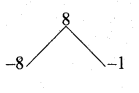# Chapter 3 – Algebra – Ex 3.7.

10 Dec 2020 2:24 pm

Tamilnadu, Samacheer, Kalvi, 10th, sslc, Maths, Solutions, Chapter 3, Algebra, Ex 3.7,

Question 1.
Find the square root of the following.
(i) \frac{400 x^{4} y^{12} z^{16}}{100 x^{8} y^{4} z^{4}}
Answer:

(ii) 7×2+214√x+2×2−12x+116
Answer:

(iii) 121(a+b)8(x+y)8(b−c)881(b−c)4(a−b)12(b−c)4
Answer:

Question 2.
Find the square root of the following
(i) 4x2 + 20x + 25
Answer:

(ii) 9x2 – 24xy + 30xz – 40yz + 25z2 + 16y2
Answer:

(iii) 1+1×6+2×3
Answer:

(iv) (4x2 – 9x + 2)(7x2 – 13x – 2)(28x2 – 3x – 1)
Answer:
4x2 – 9x +2 = 4x2 – 8x – x + 2
= 4x(x – 2)-1 (x – 2)
= (x – 2)(4x – 1)7x2 – 13x – 2 = 7x2 – 14x + x – 2
= 7x (x – 2) + 1 (x – 2)
= (x – 2) (7x + 1)28x2 – 3x – 1 = 28x2 – 7x + 4x – 1
= 7x (4x – 1) + 1 (4x – 1)
= (4x – 1) (7x + 1)(v) (2x2 + 176x + 1) (32 x2 + 4x + 2) (43 x2 + 113 x + 2)
Answer:

#### VISITORS COUNTUsers Today : 471Total Users : 87785Views Today : 1613Total views : 364932